Next: Dealing with inequalities Up: Practical implementation Previous: Practical implementation   Contents

The generic analyzer

The purpose of the generic analyzer is to consider as input any system defined in a MAPLE file and an initial set of ranges for the unknowns and to produce as output a set of ranges, strictly included in the initial set, that may contain real roots of the system. The output is presented as a file that can be used directly as input for the generic solver.

A formal-numerical approach has been used to develop this generic analyzer. Basically it takes as input a MAPLE file, called the formula file, which define the analytical form of the equations of the system and a range file in in which are defined both the names of the variables and their initial ranges (a range file may contain more than one set of ranges for the unknowns). The convention in the formula file is to define each equation of the system in the MAPLE file as a sentence prefixed by the key-word eqn where n is the number of the equation starting at 1. For example

eq1:=y**2*z+2*x*y*t-2*x-z:
eq2:=-x**3*z+4*x*y**2*z+4*x**2*y*t+2*y**3*t+4*x**2-10*y**2+4*x*z-10*y*t+2:
eq3:=2*y*z*t+x*t**2-x-2*z:
eq4:=-x*z**3+4*y*z**2*t+4*x*z*t**2+2*y*t**3+4*x*z+4*z**2-10*y*t-10*t**2+2:
is a valid formula file, describing a set of 4 equations. The range file has as many lines as unknowns and each line start by the name of the variable followed by two numbers indicating the range for the variable. For example

x -100 100
y -100 100
z -100 100
t -100 100
is a valid range file for the previous formula file. The analyzer is able to deal with infinity in the ranges: instead of using numbers you may indicate infinity or -infinity. In a first step the analyzer will use MAPLE to produce various intermediary files which are needed for the algorithm to proceed (see section 12.4.4.1). For example it will write in the /tmp/Equation file a description of the system compatible with the syntax used by the parser. Similarly you will find in the /tmp/Gradient_Equation and Hessian_Equation files a description of the gradient and hessian of the system. As soon as all the necessary files have been written, the algorithms described in the previous section will be used.

The result of the analyzer is a file (by default .result_IA) that describe the possibles ranges which may contain real roots of the system. This file may then be used as input for the solver. The analyzer will also produced the .gradient_IA file that indicate the value of the gradient matrices for each of the out ranges.

The analyzer is run by using the syntax:

Interval_Analyzer -F [formula file] [-G] [-H] -R [range file]
In this list of argument only the -F, -R are compulsory. The -G option indicates that you will use the monotonicity of the equations (evaluated through an interval evaluation of the gradient) to improve the interval evaluation of the equations. The -H indicates that you will also use Kantorovitch theorem to isolate the real roots of the equations. In that case if you have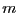equations andunknowns with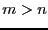, then Kantorovitch will be applied on thefirst equations.

This program will then ask you the maximum number of ranges that may be created by the algorithm, an accuracy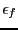on the value of the equations that will be used to stop the computation of the Newton scheme, a minimal value for the maximal diameter for the output ranges (which is not used right now), a depth level, a maximal diameter for the ranges over which Kantorovitch is not used and the value of the debug level (0 if the program is to be run quietly, 1 to see how many boxes are dealt with and 2 for a full debug). Note that if you are using the -H option and haveequations andunknowns, withand if the algorithm returns roots of the system (i.e. the output ranges are reduced to a point) it will mean that these points are guaranteed roots of thefirst equations (you may use the Newton scheme with a loweron these roots and you will still get a root), while the absolute values of the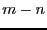remaining equations at these points will be lower thanbut there is no guarantee that these points are effectively roots of these equations.

Alternatively you may use a configuration file to give values for these parameters.

A configuration file consists in a list of keywords followed by a value.

• Result_File name: the result will be stored in the name file instead of the default .result_IA file.
• Iteration integer: the maximum number of boxes that may be produced by the algorithm. Indicating this value is compulsory.
• Debug integer: the debug level (0=no debug, 1=low level debug, 2=full debug)
• AccuracyF real: the epsilonf parameter that will be used to determine a solution if Moore or Kantorovitch succeed.
• Kanto real: we will apply Kantorovitch test as soon as the maximal width of the input ranges is lower than real
• Min_IA real, Mini_IA real: if the change in a variable exceed a threshold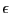we start again the analysis. We give here two values such that Mini_IA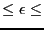Min_IA. The default value is 0.001 for Mini_IA and 0.01 for Min_IA
• Directory name: if you have already processed the equations with MAPLE you may give here the name of the directory where the files resulting from the processing have been written. This avoid to re-process the equations.
• Location_IS name: the location of the library lib_IS.m
• Level integer: the level of bisection you want (from -1, no bisection to any positive number)
• Use_Resultant: the resultant of any two equations which are algebraic in any variable will be computed and will be used to discard input ranges. This option may add a significant amount of time to the pre-preprocessing. If you have a large number of equations you will end up with a large number of equations resulting from the computation of the resultant. You may however use only a subset of these equations by first processing the equations without this option, compute some resultant equations by hand and indicate that they are resultant equation by writing them in parser format in the file /tmp/Resultant, while indicating their number in the file /tmp/DimensionResultant. If you are using the option -G or -H of the analyzer you will have to provide also the gradient equations of the equations you are using in a file called Gradient_Resultant.
• LevelC integer: if the integeris greater or equal to 0 the first test the analyzer will perform is to consider in turn each variable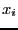and to compute the interval evaluation of the equations withhaving as interval value first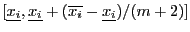and then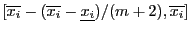. If the interval evaluation of one equation has a constant sign, then the interval forwill be modified to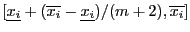in the first case and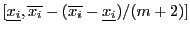in the second case. If one variable at least is modified with an amplitude of modification larger than Mini_IA the process is reiterated. Instead of using this process each time a variable is change we may use it only from time to time. This may be done by setting LevelC to a negative number lower or equal to -10. This number will indicate at the same time the number of variable changes that must occur before using the process and the value ofused for managing the interval. For example a value of -100 will indicate that the process will be used every 10 changes of variable (rint(-LevelC)/10) with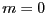(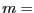10(-LevelC/10-rint(-LevelC)/10)). This process may be computer intensive and therefore the default value of LevelC is -1 so that it is not used.
• UseFullCycle: when using the bound theorems the analyzer will consider in turn each equation and then in turn each variable in which the current equation is algebraic. If an update in a variable has taken place with an amplitude larger than Mini_IA the analyzer will continue until all variables of the current equation has been considered and then will start again the analysis from equation 1. If you put this key-word in your configuration file the analyzer will start again the analysis only when all the equations have been processed. Turning on this option may be interesting if you have used the LevelC option as processing the equations is in general faster than using the combinatory approach: with this option you may have change on a larger number of unknowns which may lead to better result when using the combinatory approach.
For example

Iteration 1000
AccuracyF 0.00001
Debug 0
Kanto 0.5
Level 2
Location_IS /u/ALIAS/Maple
is a valid configuration file. It indicates that at most 1000 ranges may be created (this is not the maximal number of ranges at the end of the algorithm but the maximal number of ranges that may be created at any time in the algorithm), that the accuracy for the Newton scheme is 0.00001, that the algorithm will run quietly, that Kantorovitch will be used as soon as the maximal diameter of the considered range is lower than 0.5 and that the depth level will be 2. We indicate also in which directory is located the library lib_IS. files are located.

You use the configuration file with the syntax:

Interval_Analyzer -F [formula file] -G -H -R [range file] -C
[configuration file]
So a typical analysis and solving process will be done by using the two following command lines (which are basically identical to what is used in the script script_solver provided in the standard distribution of ALIAS):

Interval_Analyzer -F equation.maple -G -H -R range -C conf_analyzer
Interval_Solver -F /tmp/Equation -G /tmp/Gradient_Equation -H
/tmp/Hessian_Equation -R .result_IA -GI .gradient_IA -C conf_solver
You will then have the result of the solution of your system in the file .result_IS.Next: Dealing with inequalities Up: Practical implementation Previous: Practical implementation   Contents
Jean-Pierre Merlet 2012-12-20